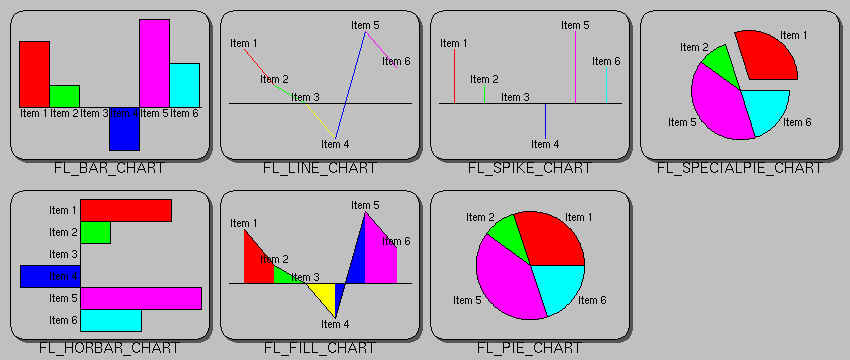# DocumentationFLTK AppsFLTK LibraryForumsLinksLoginHome  |  Articles & FAQs  |  Bugs & Features  |  Documentation  |  Download  ]

## class Fl_Chart

### Class Hierarchy

```Fl_Widget
|
+----Fl_Chart
```

### Include Files

```#include <FL/Fl_Chart.H>
```

### Description

This widget displays simple charts and is provided for Forms compatibility.### Methods

#### Fl_Chart::Fl_Chart(int x, int y, int w, int h, const char *label = 0)

Creates a new Fl_Chart widget using the given position, size, and label string. The default boxtype is FL_NO_BOX.

#### virtual Fl_Chart::~Fl_Chart()

Destroys the Fl_Chart widget and all of its data.

#### void add(double value, const char *label = NULL, uchar color = 0)

The add method adds the value and optionally label and color to the chart.

#### uchar autosize(void) const void autosize(uchar onoff)

The autosize method controls whether or not the chart will automatically adjust the bounds of the chart. The first form returns a boolean value that is non-zero if auto-sizing is enabled and zero is auto-sizing is disabled.

The second form of autosize sets the auto-sizing property to onoff.

#### void bounds(double *a, double *b) void bounds(double a, double b)

The bounds method gets or sets the lower and upper bounds of the chart values to a and b respectively.

#### void clear(void)

The clear method removes all values from the chart.

#### void insert(int pos, double value, const char *label = NULL, uchar color = 0)

The insert method inserts a data value at the given position pos. Position 1 is the first data value.

#### int maxsize(void) const void maxsize(int n)

The maxsize method gets or sets the maximum number of data values for a chart. If you do not call this method then the chart will be allowed to grow to any size depending on available memory.

#### void replace(int pos, double value, const char *label = NULL, uchar color = 0)

The replace method replaces data value pos with value, label, and color. Position 1 is the first data value.

#### int size(void) const

The size method returns the number of data values in the chart.

#### uchar type() const void type(uchar t)

The first form of type() returns the current chart type. The chart type can be one of the following:
FL_BAR_CHART
Each sample value is drawn as a vertical bar.
FL_FILLED_CHART
The chart is filled from the bottom of the graph to the sample values.
FL_HORBAR_CHART
Each sample value is drawn as a horizontal bar.
FL_LINE_CHART
The chart is drawn as a polyline with vertices at each sample value.
FL_PIE_CHART
A pie chart is drawn with each sample value being drawn as a proportionate slice in the circle.
FL_SPECIALPIE_CHART
Like FL_PIE_CHART, but the first slice is separated from the pie.
FL_SPIKE_CHART
Each sample value is drawn as a vertical line.
The second form of type() sets the chart type to t.